# 2nd PUC Chemistry Previous Year Question Paper June 2018

Students can Download 2nd PUC Chemistry Previous Year Question Paper June 2018, Karnataka 2nd PUC Chemistry Model Question Papers with Answers helps you to revise the complete Karnataka State Board Syllabus and score more marks in your examinations.

## Karnataka 2nd PUC Chemistry Previous Year Question Paper June 2018

Time: 3:15 Hours
Max. Marks: 70

Instructions

• The question paper has four parts A, B, C, and D. All parts are compulsory.
• Write balanced chemical equations and draw labeled diagrams wherever required.
• Use Jog tables and simple calculators if necessary (Use of scientific calculators is not allowed).

Part – A

I. Answer all the following questions: (10 × 1 = 10)

Question 1.
In a binary solution, the mole fraction of one component is 0.068. What is the mole fraction of another component?
0.932.

Question 2.
State Henry’s law.
At constant temperature, the solubility of a gas in a liquid is directly proportional to the partial pressure of the gas present above the surface of the solution.
p = KH. x
p: partial pressure of the gas.
x: mole fraction of the gas.
KH: Henry’s constant.Question 3.
Why does the conductivity of a solution decrease with dilution?
The number of ions per unit volume that carries the current in a solution decreases.

Question 4.
A chemical reaction has the rate expression, Rate = K[A]2 [B]. What is its overall order?
3.

Question 5.
Give the principle involved in the zone refining process.
The impurities are more soluble in the melt than in the solid state of the metal.

Question 6.
Which noble gas does not occur in the atmosphere?

Question 7.
What is the value of the co-ordination number of Fe in K[Fe(CN)6]?
6Question 8.
In aryl halides, what is the hybridization of the carbon atom to which halogen is attached?
Sp2.

Question 9.
Write the IUPAC name of CH3COCH2CH2CH3.
Pentan-2-one.

Question 10.
Name the nitrogen base present only in DNA, not in RNA.
Thymine.

Part – B

Answer any FIVE questions (Each question carries two marks): (5 × 2 = 10)

Question 11.
Calculate the number of particles (atoms) per unit cell in an FCC crystal lattice.
No. of particles (atoms) in FCC unit cell
= $$\frac {1}{8}$$ × 8 (at corners) + $$\frac {1}{2}$$ × 6 (at faces)
= 1 + 3
= 4 particles.

Question 12.
Draw a neat labeled diagram of H2 – O2 fuel cell and write overall cell reaction.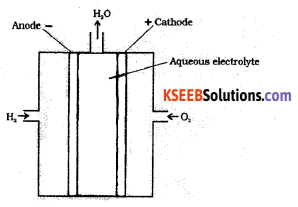In this cell, hydrogen and oxygen are bubbled through porous carbon electrodes into concentrated aqueous sodium hydroxide.
Hydrogen is fed into the anode compartment where it is oxidized.
The oxygen is fed into the cathode compartment where it is reduced.Question 13.
The rate constant of a first-order reaction is 1.15 × 10-3 s-1. Calculate its half-life period (t½).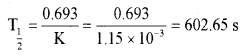Question 14.
Give reason:

1. What is lanthanoid contraction?
2. Which is the general oxidation state shown by actinoids?

1. Radii of members of 3 transition series are very much similar to corresponding members of 2 series.
2. Very difficult to separate lanthanoids of 2 series elements from 3rd series elements in the pure state during extraction.Question 15.
How does phenol react with cone, nitric acid? Give an equation.
(i) With dilute nitric acid at low temperature (298 K) phenol yields a mixture of ortho and para nitrophenols.(ii) With Conc. nitric acid phenol is converted to 2, 4, 6-trinitrophenol (picric acid).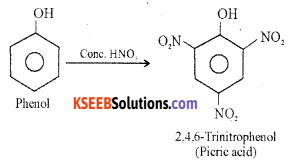Question 16.
Explain Cannizzaro’s reaction to an example.
Aldehydes which do not have an α-hydrogen atom, undergo self-oxidation and reduction reaction on heating with conc. alkali. In this reaction, one molecule of the aldehyde is reduced to alcohol while another is oxidized to carboxylic acid salt.Question 17.
What are analgesics? Give one example for non-narcotic analgesics.
Analgesics are chemicals used to reduce body pain without causing impairment of consciousness.
Ex: Non-norcotic analgesic – Aspirin;
Norcotic analgesic – Morphine.Question 18.
What is saponification? Give the equation to form sodium stearate by this method.
Soap is prepared by a heating aqueous solution of potassium or sodium hydroxide with fat or oil. This reaction is called saponification.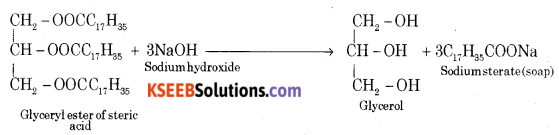Part – C

Answer any FIVE of the following (Each question carries three marks): (5 × 3 = 15)

Question 19.
Draw a neat labeled diagram of electrolytic cells used in the extraction of Aluminium by the Hall-Heroult process. Write the reactions that take place at cathode and anode.
Purified alumina is fused with cryolite and fluorspar which lowers the melting point of the alumina. The fused mass is taken in steel vessels lined with carbon lining acts as a cathode. Bunch of carbon rods dipped in the molten solution acts as an anode.On electrolysis aluminum is discharged at cathode and oxygen is liberated at the anode which reacts with the carbon of the anode a producing CO and CO2.
The electrolytic reactions are:
Cathode: Al3+ + 3e → Al
Anode: C + O2- → CO + 2e
C + 2O2- → CO2 + 4e

Question 20.
Write the equations with conditions for the manufacture of nitric acid by Ostwald’s process.
Step 1: Catalytic oxidation of ammonia gives nitric oxide.Step 2: Nitric oxide thus formed combines with oxygen gives NO2Step 3: Nitrogen dioxide so formed diso1ves in water to give HNO3
H2O + 3NO2 → 2HNO3 + NOQuestion 21.
Complete the following equations:
(i) SO2 + Cl2 $$\underrightarrow { charcoal }$$ ____
(ii) S + 2H2SO4 → _____
(iii) NO + O3(conc.) → _____
(i) SO2 + Cl2 $$\underrightarrow { charcoal }$$ SO2Cl2 (Sulphuryl Chloride)
(ii) S + 2H2SO4 → 2H2O + 3SO2
(iii) NO + O3 → NO2 + O2

Question 22.
(a) Write any two anomalous properties of fluorine. (2)
The anomalous behavior of fluorine is due to its

• Smaller size.
• Highest electronegativity.
• Low F — F bond dissociation enthalpy.
• Non-availability of d-orbitals in the valence shell.

(b) Give an equation for the reaction of chlorine with hydrogen sulphide. (1)
It reacts with the compounds containing hydrogen to form HCl.
H2 + Cl2 → 2HCl
H2S + Cl2 → 2HCl + S
C10H16 + 8Cl2 → 16HCl + 10C

Question 23.
(a) Transition metals show catalytic property: Give reasons. (2)
Interstitial compounds are those which are formed by transitional element. when small atoms like H, C, and N are trapped inside the crystal lattices of metals.
Example: VH0.5

(b) Between $$\mathbf{C u}_{(\mathbf{a q})}^{\mathbf{2 +}}$$ and $$\mathbf{C u}_{(\mathbf{a q})}^{+}$$ which is more stable? (1)
Cu2+Question 24.
Write the balanced equations in the manufacture of potassium dichromate from chromite ore.
The chromate and dichromates are interconvertible in aqueous solutions depending upon the pH of the solution.
At low pH (acidic medium):
Chromate converts into dichromateAt high pH (alkaline medium):
dichromate is converted into chromate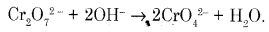Question 25.
Explain the hybridization, geometry, and magnetic property of [CoF6]3- ion on the basis of the Valence Bond Theory (Atomic number of Co = 27).
(i) Orbitals of Co3+ ion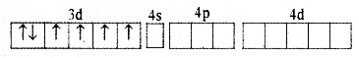(ii) In Co3+ ion sp3d2 hybridization takes place d2 hybridized orbitals of Co3+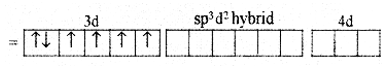(iii) Six pairs of electrons, one from each F- ion occupy the six hybrid orbitals. Thus, the complex has octahedral geometry and paramagnetic in nature due to the presence of four unpaired electrons.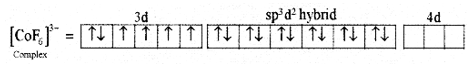For [CoF6]3- Complex:

• Type of hybridisation: sp3d2
• Geometry: Octahedral
• Magnetic property: Parainagnetic.

Question 26.
(a) What is an ambidentate ligand? Name the type of structural isomerism arises when such ligand present in the complex.
Ligand which can ligate through two different atoms is called ambidentate ligand.(b) Write the IUPAC name of K2[Zn(OH)4].
K2[Zn(OH)4] – Potassium tetrahydraxidozincate(II)

Part – D

IV. Answer any three of the following (Each question carries five marks): (3 × 5 = 15)

Question 27.
(a) Calculate the packing efficiency in a body-centered cubic lattice.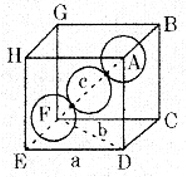From the figure, it is clear that the atom at the center will be in touch with the other two atoms along the body diagonal.
Let a: be the edge length of the cube
b: be the face diagonal
c: be the body diagonal
r: is the radius of the sphere and c = 4r
In ∆EFD
b2 = a2 + a2
b2 = 2a2
In ∆AFD
c2 = a2 + b2
c2 = a2 + 2a2 = 3a2
c = √3 × a
4r = √3 × a
a = $$\frac{4 r}{\sqrt{3}}$$
Volume of the cube = $$a^{3}=\left(\frac{4}{\sqrt{3}} r\right)^{3}$$
In this unit cell, total number of particles is 2 and volume is 2 × $$\frac{4}{3} \pi r^{3}$$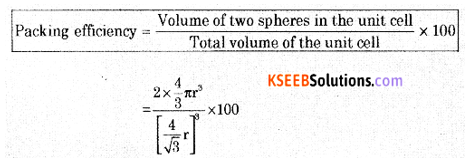Packing efficiency = 68%

(b) What is a Schottky defect?
It is a type of defect that arises in ionic solids in which an equal number of cations and anions are missing from their lattice points. This defect decreases the density of the ionic solid.

Question 28.
(a) 5.8g of non-volatile, non-electrolyte solute was dissolved in 100 g of carbon disulphide (CS2). The vapor pressure of the solution was found to be 190 mm of Hg. Calculate the molar mass of the solute.
Given: Vapour pressure of pure CS is 195 mm of Hg and the molar mass of CS2 is 76g/mol.
Data: Vapour pressure of solvent = P0 = 195mm
Vapour pressure of solution = P = 190 mm
P0 – P = 195 – 190 = 5 mm
Relative lowering of vapour pressure = $$\frac{P^{\circ}-P}{P}=\frac{5}{195}$$ = 0.02564
Mass of solute in grams = W2 = 5.8g
Mass of solvent (CS2) in grams = 100g
Molar mass of solvent (CS2) = 76g mol-1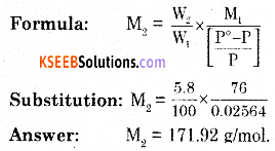(b) Mention any two differences between ideal and non-ideal solutions.
The ideal solution
(i) Obey’s Raoult’s law over the entire range of concentration.
(ii) There is no change in enthalpy when the solution is formed from its components.
ΔHmax = 0
(iii) There is no change in volume when the solution is formed from its components.
ΔVmax = 0
(iv) The interactions between the components in solution are similar to those in the pure components.Non-ideal solution
(i) Does not obey Raoult’s law over the entire range of concentration.
(ii) There is a change in enthalpy when the solution is formed from its components.
ΔHmax ≠ 0
(iii) There is a change in volume when the solution is formed from its components.
ΔVmax ≠ 0
(iv) The interaction between the components in solution is different from those of the pure components.

Question 29.
(a) Calculate e.m.f of the cell in which the following reaction takes place: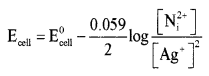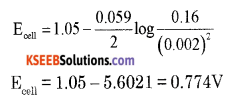(b) State the Faraday’s first law of electrolysis. How many Faraday of electricity is required for the reduction of 1 mole of Mg2+ ions?
During electrolysis, the amount of any substance deposited or liberated at an electrode is directly proportional to the quantity of electricity passed through the electrolytic solution.
W = ZQ; Q = It
W = ZIt
W → Mass of substance discharged at an electrode.
Z → Electrochemical equivalent.
I → Electric current in amperes.
t → Time in seconds.
2 Faraday’s of electricity is required.Question 30.
(a) Derive an integrated rate equation for the rate constant of a zero-order reaction.
Consider a zero-order reaction.
R → P
Rate = $$\frac{-d[R]}{d t}$$ = k[R]0
where k is rate constant for zero order reaction.
$$\frac{-d[R]}{d t}$$ = k
d[R] = -k.dt
Integrating both sides
∫d[R] = -∫k.dt
[R] = -kt + I ….. (1)
I is constant in integration.
At t = 0, [R] = [R]0
where [R]0 is initial concentration of the reactant.
Substituting in equation (1)
[R]0 = -k × 0 + I
I = [R]0
Substituting I value in equation (1)
[R] = -kt + [R]0
$$k=\frac{[R]_{0}-[R]}{t}$$

(b) Draw a graph showing effect of catalyst on the activation energy of a chemical reaction.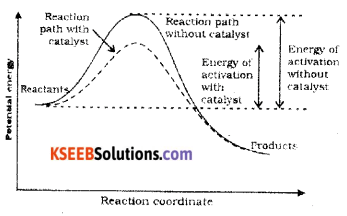Catalyst provides an alternate pathway by reducing the activation energy and lowering the energy barrier. More molecules enter the threshold energy state leading to chemical reaction and hence increases the rate.

Question 31.
(a) Write any two characteristics of chemical adsorption.
When the molecules of the adsorbate are held to the surface of the adsorbent by the chemical bonds, the adsorption is called chemical adsorption or chemisorption.
Characteristics:

• It is specific in nature.
• It is irreversible in nature.
• Adsorption increases as temperature increases.

(b) What is the Brownian movement? What is the cause of it?
In the dispersion medium, colloidal particles are in continuous zig random motion in all directions. This type of kinetic motion of the colloidal particles is called the Brownian movement.
Brownian movement is due to the bombardment between the colloidal particles and the particles of the dispersion medium.(c) What is homogeneous catalysis?
A catalytic process in which reactant and the catalyst are in the same phase, then the process is called homogeneous catalysis.V. Answer any four of the following (Each question carries five marks): (4 × 5 = 20)

Question 32.
(a) Write equations for the steps in the SN1 mechanism of conversion of tertiary butyl bromide into tertiary butyl alcohol.
The reaction between tert-butyl bromide and hydroxide ion gives tert-butyl alcohol. The rate of reaction depends only on the concentration of tert-butyl bromide. Hence it is a nucleophilic first order substitution reaction.Mechanism:
Step 1: Polarised C — Br undergoes cleavage to produce planar carbocation.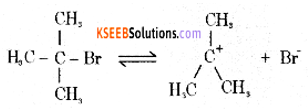Step 2: The carbocation is then attacked by a nucleophile (OH) on either side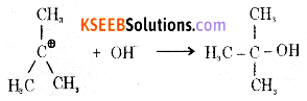(b) Explain the Wurtz-Fittig reaction with the equation.
A mixture of an alkyl halide and aryl halide gives an alkyl arene when treated with sodium in dry ether.(c) CH3Cl + Nal $$\underrightarrow { Acetone }$$ CH3l + NaCl. Name the above reaction.
Finkelstein Reaction

Question 33.
(a) Write the mechanism of acid-catalyzed dehydration of ethanol to ethene.
Step 1: Formation of protonated alcoholStep 2: Formation of carbocation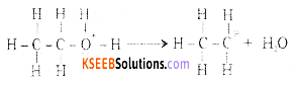Step 3: Formation of ethene by the elimination of a proton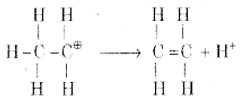(b) How does anisole react with bromine in ethanoic acid? Give equation.
Anisole undergoes bromination with bromine in ethanoic acid to form orthobromoanisole and para-bromoanisole.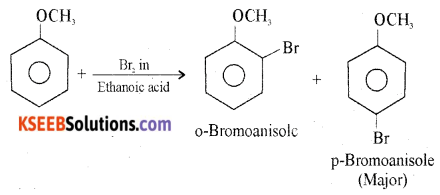Question 34.
(a) Complete the following equations: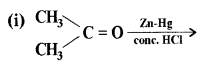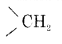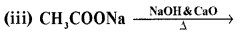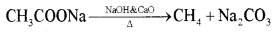(b) Explain the esterification reaction with an example.
Alcohols react with carboxylic acids, in the presence of Conc. H2SO4 to form esters. This reaction is known as esterification.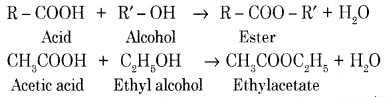Question 35.
(a) How is methenamine prepared by Hoffmann bromamide degradation reaction? Give equation.
When ethanamide (acetamide) is treated with bromine in an aqueous solution of sodium hydroxide, methylamine is obtained.
CH3CONH2 + Br2 + 4NaOH → CH3NH2 + 2NaBr + 2H2O + Na2CO3

(b) How is aniline converted to Benzene diazonium chloride? Give equation.
The conversion of primary aromatic amines into diazonium salts is known as diazotization.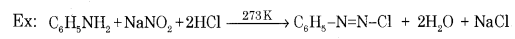(c) Between ammonia and aniline, which is more basic?
Ammonia.

Question 36.
(a) Write the Haworth structure of Maltose.(b) What are non-essential amino acids? Name naturally occurring a-amino acid which is not optically active.
Amino acids that are synthesized in the body are known as non-essential amino acids.
Example: Glycine, Alanine.
Amino acids that are not synthesized in the body and must be supplied through the diet are known as essential amino acids.
Example: Lysine, Histidine.
Glycine

(c) Which vitamin deficiency causes the disease ‘scurvy’?
Vitamin C.Question 37.
(a) How is nylon 6, 6 prepared? Give equation.
It is obtained by the condensation polymerization of hexamethylene diamine with adipic acid under high pressure and at high temperature.(b) Write the partial structure of
(i) Polythene